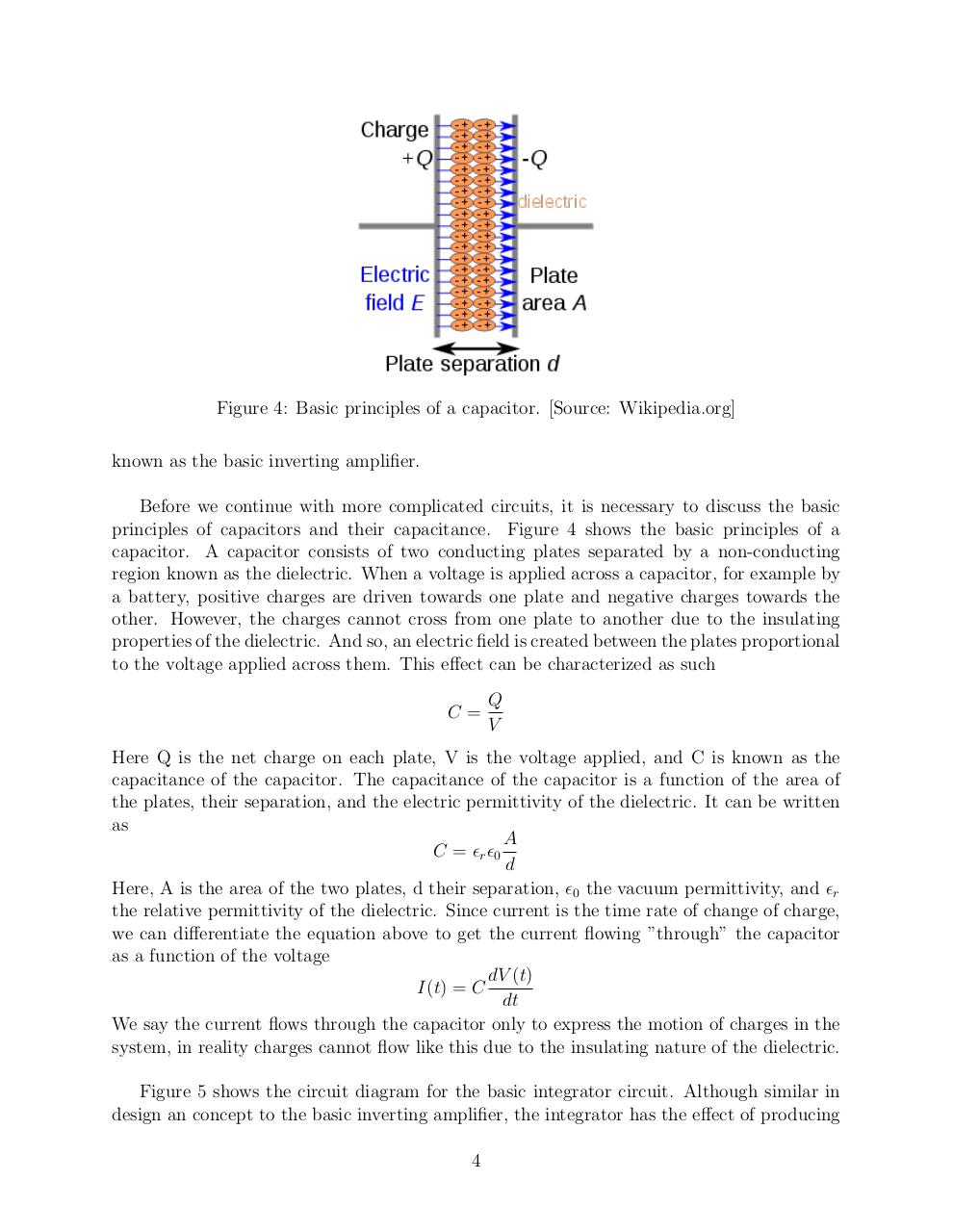# Op Amps.pdfPage 1 2 3 4 5 6 7 8 9 10 11 12 13

#### Text preview

Figure 4: Basic principles of a capacitor. [Source: Wikipedia.org]
known as the basic inverting amplifier.
Before we continue with more complicated circuits, it is necessary to discuss the basic
principles of capacitors and their capacitance. Figure 4 shows the basic principles of a
capacitor. A capacitor consists of two conducting plates separated by a non-conducting
region known as the dielectric. When a voltage is applied across a capacitor, for example by
a battery, positive charges are driven towards one plate and negative charges towards the
other. However, the charges cannot cross from one plate to another due to the insulating
properties of the dielectric. And so, an electric field is created between the plates proportional
to the voltage applied across them. This effect can be characterized as such
C=

Q
V

Here Q is the net charge on each plate, V is the voltage applied, and C is known as the
capacitance of the capacitor. The capacitance of the capacitor is a function of the area of
the plates, their separation, and the electric permittivity of the dielectric. It can be written
as
A
C = r 0
d
Here, A is the area of the two plates, d their separation, 0 the vacuum permittivity, and r
the relative permittivity of the dielectric. Since current is the time rate of change of charge,
we can differentiate the equation above to get the current flowing ”through” the capacitor
as a function of the voltage
dV (t)
I(t) = C
dt
We say the current flows through the capacitor only to express the motion of charges in the
system, in reality charges cannot flow like this due to the insulating nature of the dielectric.
Figure 5 shows the circuit diagram for the basic integrator circuit. Although similar in
design an concept to the basic inverting amplifier, the integrator has the effect of producing
4